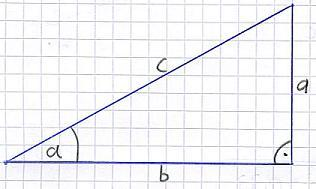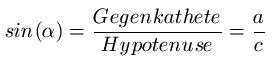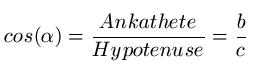# How does a sine calculator work

## Sine, cosine and tangent (trigonometric functions)

In this section on trigonometry we show you how you can calculate angles with sine, cosine / cosine and tangent. You will get to know terms such as adjacent, opposite and hypotenuse. In addition to explanations and examples, you will also find exercises to help you cope better with the content.

The sine, cosine and tangent functions for calculating an angle may only be used on a right-angled triangle. The following graphic shows you such a triangle. You can find more information below:Sine, Cosine and Tangent (Trigonometric Functions) Video:

Show:

So much for a triangle. At this point you have to remember a few terms. These appear again and again in the calculation. There are a few properties to note:

• On the right, at the bottom of the triangle, a right angle has been drawn
• We denote the angle at the bottom left as α (spoken: Alpha)
• The side "a" is referred to as the opposite cathetus because it lies opposite the angle α
• The side "b" is called the adjacent because it lies at the angle α
• The side "c" is called the hypotenuse

You should already be familiar with the terms adjacent, opposite and hypotenuse from the Pythagorean theorem. With this knowledge we can now calculate angles and - if the angle is given - lengths.

Show:

### Sine

Time to count. We start with the sine. The following mathematical relationship applies:Remarks:

• An angle in degrees is used for alpha (α), for example 20 degrees or 40 degrees.
• The lengths for the opposite cathetus and hypotenuse must be used in the same units, for example, insert everything in meters.
• You have to set your calculator to DEG (Degree), otherwise you will get a wrong result.
• If you want to calculate the angle, you have to work with arcsin (see examples)

Example 1:
The opposite cathetus has a length of 3 cm (a = 3 cm) and the hypotenuse has a length of 5 cm (c = 5 cm). How big is the angle α (alpha)?

Table scrollable to the right
 Solution: sinα = a: c sinα = 3cm: 5cm sinα = 0.6 | arcsin α = 36.87 degrees

Plug the numbers into the sine equation. Then the division is calculated on the right-hand side. You get sinα = 0.6. Now comes the interesting part: To get rid of the sin, you have to use arcsin. So you have to go into the calculator arcsin 0.6 enter. This results in an angle of 36.87 degrees (if you set your calculator to Degree).

### Cosine / cosine

After the sine we come to the cosine / cosine. The formula looks like this:Remarks:

• An angle in degrees is used for alpha (α), for example 25 degrees or 45 degrees.
• The lengths for the adjacent and hypotenuse must be used in the same units, for example, insert everything in meters.
• You have to set your calculator to Degree, otherwise you will get a wrong result.
• If you want to calculate the angle, you have to work with arccos (see examples)

Example 2:

The adjacent cathetus has a length of 3 cm (b = 3 cm) and the hypotenuse has a length of 5 cm (c = 5 cm). How big is the angle α (alpha)?

Table scrollable to the right
 solution cosα = b: c cosα = 3cm: 5cm cosα = 0.6 | arccos α = 53.13 degrees

Plug the numbers into the cosine equation. Then the division is calculated on the right-hand side. You get cosα = 0.6. Now comes the interesting part: To get rid of the cos, you have to use arccos. So you have to go into the calculator arccos 0.6 enter. This results in an angle of 53.13 degrees (if you set your calculator to Degree).

### tangent

After sine and cosine, the tangent function is now on. Here, too, first of all the formula:Remarks:

• An angle in degrees is used for alpha (α), for example 30 degrees or 50 degrees.
• The lengths for the opposite and adjacent cathets must be used in the same units, for example everything in meters.
• You have to set your calculator to DEG (Degree), otherwise you will get a wrong result.
• If you want to calculate the angle, you have to work with arctan (see examples)

Example 3:

The adjacent cathete has a length of 3 cm (b = 3 cm) and the opposite cathete has a length of 3 cm (a = 3 cm). How big is the angle α (alpha)?

Table scrollable to the right
 solution tanα = a: b tanα = 3cm: 3cm α = 45 degrees

Plug the numbers into the tangent equation. Then the division is calculated on the right-hand side. You get tanα = 1. Now comes the interesting part: To get rid of the tan, you have to use arctan. So you have to go into the calculator arctan 1.0 enter. This results in an angle of 45 degrees (if you set your calculator to Degree).

Left:

### Who's Online

We have 407 guests online